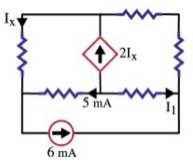# Problem: Given: The circuit below. Find I1 using KCL.

###### FREE Expert Solution

This problem is based on Kirchhoff's junction rule.

At a junction:

$\overline{){\mathbf{\Sigma }}{{\mathbf{i}}}_{\mathbf{i}\mathbf{n}}{\mathbf{=}}{\mathbf{\Sigma }}{{\mathbf{i}}}_{\mathbf{o}\mathbf{u}\mathbf{t}}}$

95% (37 ratings)###### Problem Details

Given: The circuit below. Find I1 using KCL.